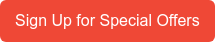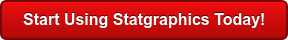# Instructional Videos

This page presents a series of statistics videos created by the developers of STATGRAPHICS Centurion, designed to help you get the most value from the program. These online statistics tutorials are presented by Dr. Neil Polhemus, the original creator of STATGRAPHICS more than 30 years ago and current CTO of Statpoint Technologies Inc. The statistics videos will teach you not only how to use the program but also many of the statistical concepts on which the procedures are built. If there are other topics you'd like to see discussed in these statistics videos, please contact support@statgraphics.com for suggestions on our online statistics tutorials.WEBINARS
A large collection of recorded webinars are available on our Webinars page.

STATGRAPHICS 19
These videos demonstrate the new features contained in Version 19.

OVERVIEW
Statgraphics 19 Additions and Enhancements (13:48)

USER INTERFACE
Interface to Python (14:12)
Using the Statgraphics Dashboard (8:23)

SPC AND DESIGN OF EXPERIMENTS
Conformance Analysis (6:27)
Constructing Alias Optimal  Designs (12:50)
Gage R&R Studies (GLM Method) (7:37)
Optimizing Existing Designs (6:11)

STATISTICAL MODELING
Equivalence and Non-Inferiority Tests for Variance (11:12)
Fitting Bivariate Mixture Distributions (5:07)
Fitting Univariate Mixture Distributions (8:08)
Fitting Johnson Distributions (7:33)
GLM Stepwise Effect Selection (8:35)
Piecewise Linear Regression (9:05)
Quantile Regression (8:35)
Stability Studies (6:46)
Zero-Inflated Count Distributions (6:07)
Zero-Inflated Count Regression (17:11)

MACHINE LEARNING
Decision Forests (17:30)
K-Means Clustering (12:02)

DATA VISUALIZATION
Barcharts with Lines (1:48)
Venn and Euler Diagrams (9:50)
Waterfall Plots (7:50)

STATGRAPHICS 18
These videos demonstrate the new features introduced in the latest version of our Windows desktop product, featuring over 260 statistical procedures.

OVERVIEW

GETTING STARTED

Step 1: (8:51)
Step 2: (4:20)
Step 3: Activation:
(3:33)
Check-out/check-in seats (7:40)

BIG DATA

DATA MINING & MACHINE LEARNING

DATA VISUALIZATION

Diamond Plots (4:06)
(6:40)
Dynamic Demographic Maps (7:46)
Heat Maps (6:42)
(5:42)
Population Pyramid Statlet (4:21)
Sunflower Plots (5:03)
Trivariate Density Estimation (4:04)
Violin Plots (7:36)
Wind Rose Statlet (3:46)

DESIGN OF EXPERIMENTS

(3:24)
Definitive Screening Designs (10:47)
Ribbon Plots (3:09)

ESTIMATION AND TESTING

SIMULATION

STATISTICAL PROCESS CONTROL

TIME SERIES ANALYSIS

UTILITIES### VERSION 17 STATISTICS VIDEOS

OVERVIEW OF THE ONLINE STATISTICS TUTORIALS

NEW USER INTERFACE FEATURES
Add Objects to a Graph (6:53)
Audit Trails and StatFolio Passwords (8:03)
Creating Header Columns in a Datasheet (1:59)
Highlighting Subsets on a Graph (2:50)
Improved Axis Scaling (6:33)
Record a Video (5:18)
Tabular Options (4:34)
Using the StatLog (6:21)
Value Labels (4:05)
Zoom and Pan (4:49)

STATISTICS TUTORIALS ONLINE FOR NEW DATA VISUALIZATION
Corrgram (Correlation Plot) (5:54)
Demographic Map Brushing (8:36)
Demographic Map Visualiser (5:05)
Deviation Dashboard (7:37)
Interactive Histogram (3:14)
Multivariate Visualizer (13:42)
Open-High-Low-Close Candlestick Plot (15:35)
Probabilistic Fractal (7:04)
1D Visualizer (10:56)
2D Visualizer (8:21)
3D Visualizer (7:53)

NEW STATISTICAL ANALYSES
Bivariate Density Statlet (6:07)
Comparison of Heterogeneous Samples (9:23)
Computer Generated Optimal Designs (16:13)
Control Charts for Overdispersed Rates and Proportions (10:48)
Control Charts for Rare Events (10:24)
Curve-Fitting Statlet (11:42)
Exponential Smoothing Statlet (7:42)
Factorial Repeated Measures Designs (17:05)
Factorability Tests (12:08)
Item Reliability Analysis (6:51)
Kriging (11:45)
MIL-STD 1916 and  ANSI Z1.4 Acceptance Sampling for Attributes (14:39)
MIL-STD 1916 and  ANSI Z1.9 Acceptance Sampling for Variables (13:40)
Oneway Repeated Measures Designs (15:58)
Power Transformations Statlet (10:26)
Process Capability Analysis Statlet (31:33)
Reliability Demonstration Test Plans (9:03)
Sample Size Determination Statlet (8:19)
Surface-Fitting Statlet (10:45)
Ternary Plot (8:51)

### LEARNING CENTER SECTIONS FOR ONLINE STATISTICS TUTORIALS

STATISTICS TUTORIALS ONLINE FOR GETTING STARTED

DATA MANAGEMENT

BASIC PROGRAM OPERATION

TIPS, TRICKS, AND OTHER TIME-SAVERS

ANALYZING A SINGLE COLUMN OF NUMERIC DATA

WHAT WAS NEW IN VERSION XVI?

USING THE DESIGN OF EXPERIMENTS WIZARD

QUALITY ASSURANCE AND SIX SIGMA ONLINE STATISTICS TUTORIALS

THINGS YOU WON'T FIND IN MINITAB AND/OR JMP (in addition to all that's new in Versions 17 and 18)